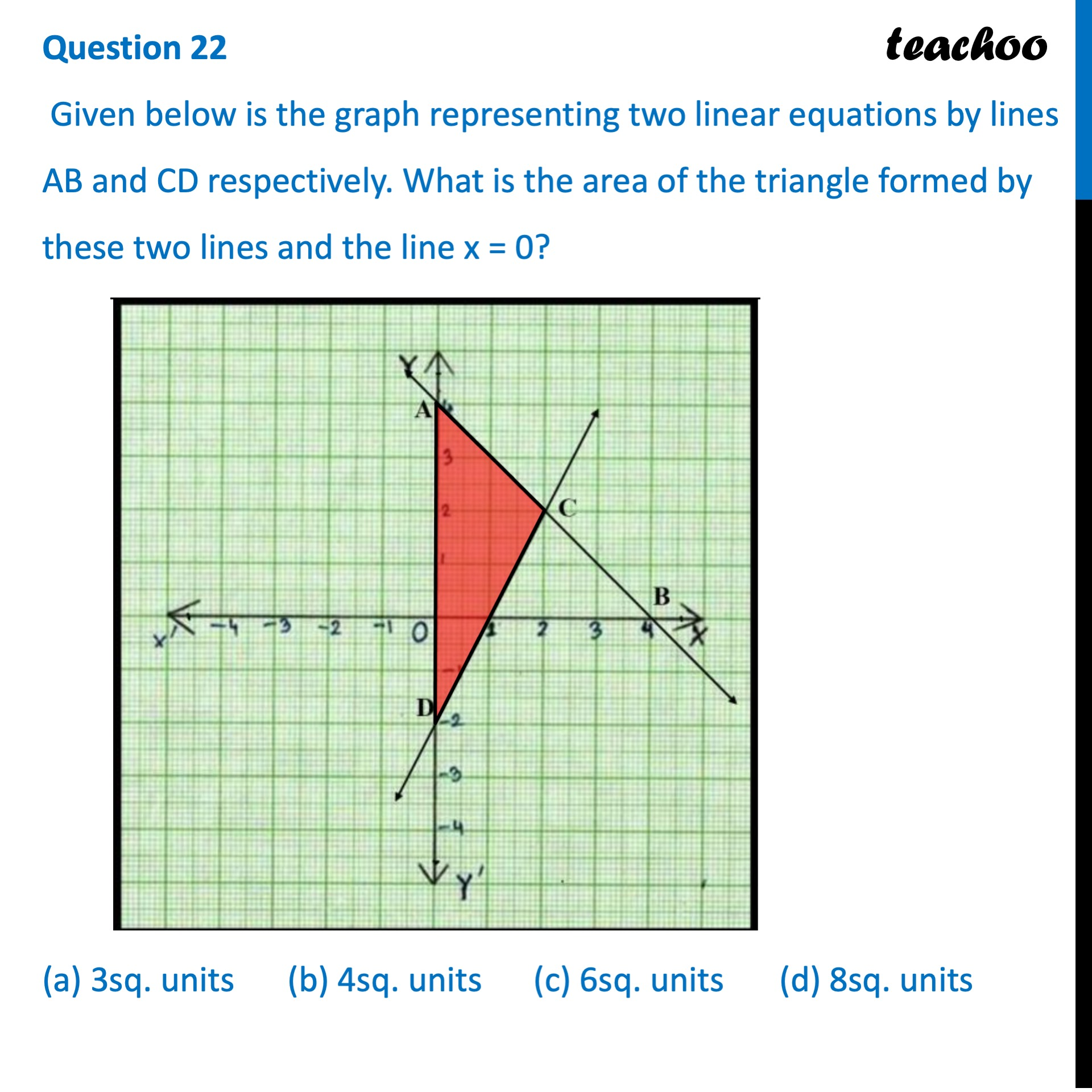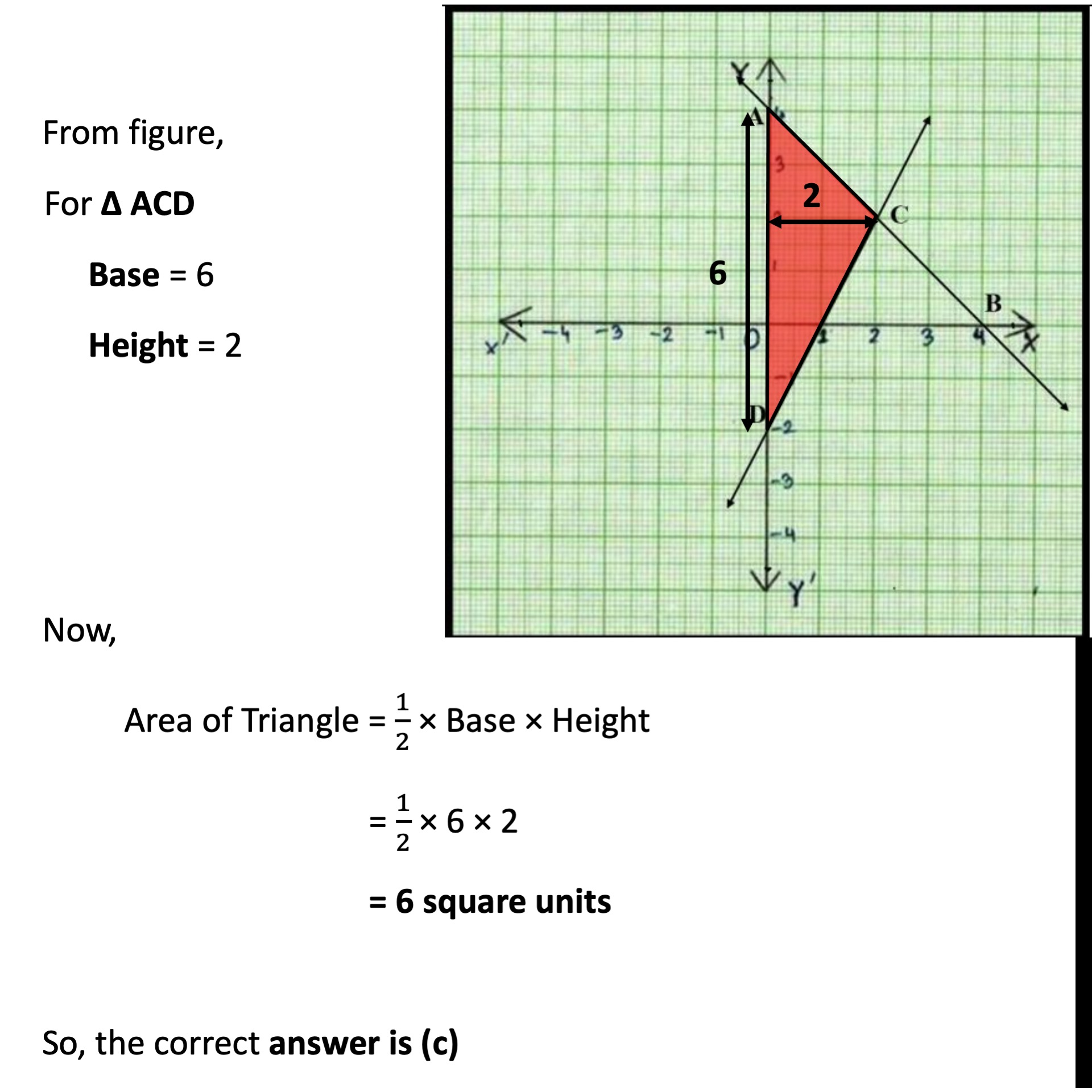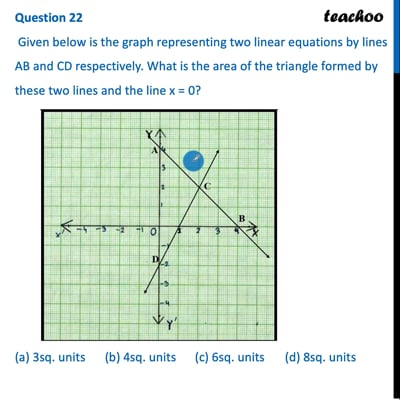CBSE Class 10 Sample Paper for 2022 Boards - Maths Standard [MCQ]

Class 10
Solutions of Sample Papers for Class 10 Boards

## (a) 3sq. units   (b) 4sq. units   (c) 6sq. units   (d) 8sq. unitsThis video is only available for Teachoo black users

Introducing your new favourite teacher - Teachoo Black, at only ₹83 per month

### Transcript

Question 22 Given below is the graph representing two linear equations by lines AB and CD respectively. What is the area of the triangle formed by these two lines and the line x = 0? (a) 3sq. units (b) 4sq. units (c) 6sq. units (d) 8sq. units From figure, For Δ ACD Base = 6 Height = 2 Now, Area of Triangle = 1/2 × Base × Height = 1/2 × 6 × 2 = 6 square units So, the correct answer is (c)